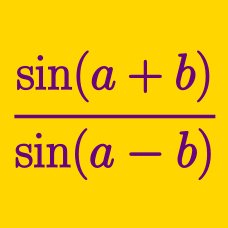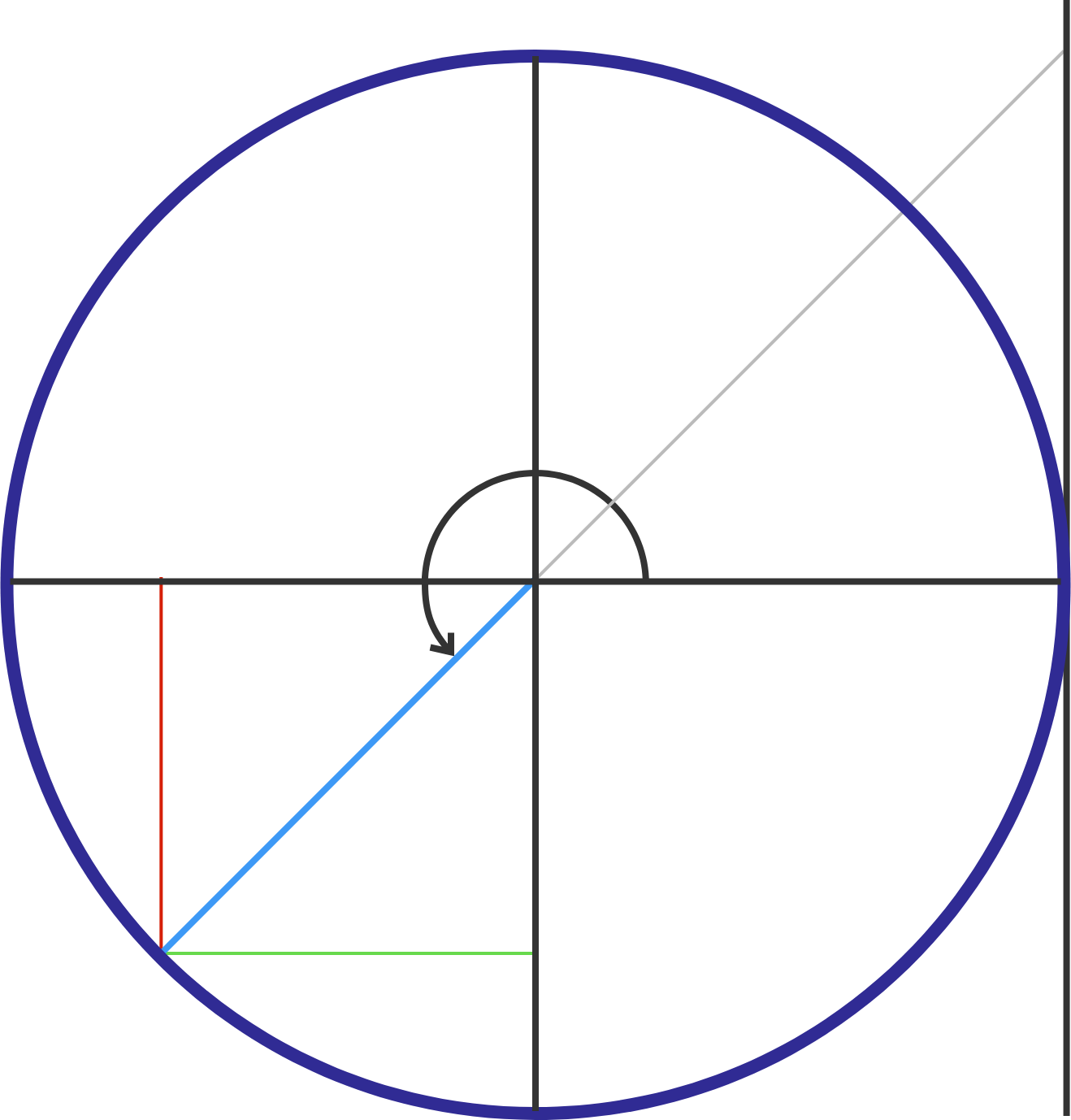Geometry

# Sum and Difference Trigonometric Formulas: Level 2 Challenges

If $$\sin\theta= \cos\theta$$, find the value of $$\cos 2\theta$$.If $$A + B = 225^{\circ}$$, find $$(1+\tan A)(1+\tan B),$$ where defined.

$\large \frac {1}{\cos 80^\circ } - \frac {\sqrt 3}{\sin 80^\circ } = \ ?$

Hint: How can we write the numerators in relation to common trig values?

If $\cos\left[6\arccos \left(\dfrac{1}{3}\right)\right] = \frac{A}{B},$ where $$A$$ and $$B$$ are relatively prime positive integers, find $$B-A.$$

$\sin^2 18^ \circ + \sin ^2 30 ^ \circ.$

Which of the following is equal to the above expression?

×

Problem Loading...

Note Loading...

Set Loading...